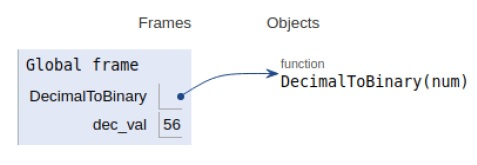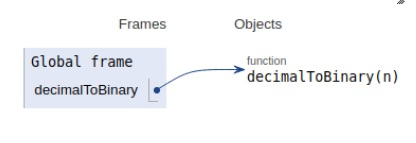# Convert decimal to binary number in Python program

Problem statement − We are given a decimal number, we need to convert it into its binary equivalent.

There are two approaches to solve the given problem. Let’s see them one by one−

## Recursive Approach

### Example

Live Demo

def DecimalToBinary(num):
if num > 1:
DecimalToBinary(num // 2)
print(num % 2, end = '')
# main
if __name__ == '__main__':
# decimal input
dec_val = 56
# binary equivalent
DecimalToBinary(dec_val)

### Output

111000All the variables and functions are declared in the global scope shown in the figure above.

## Using Built-In method

### Example

Live Demo

def decimalToBinary(n):
return bin(n).replace("0b", "")
# Driver code
if __name__ == '__main__':
print(decimalToBinary(56))

### Output

111000All the variables and functions are declared in the global scope shown in the figure above.

## Conclusion

In this article, we have learned about the python program to convert a list into a string.